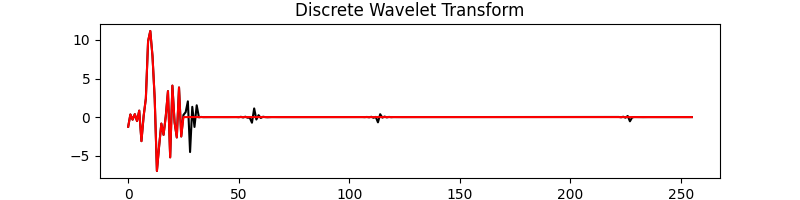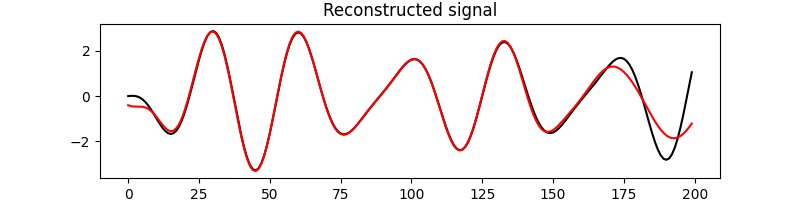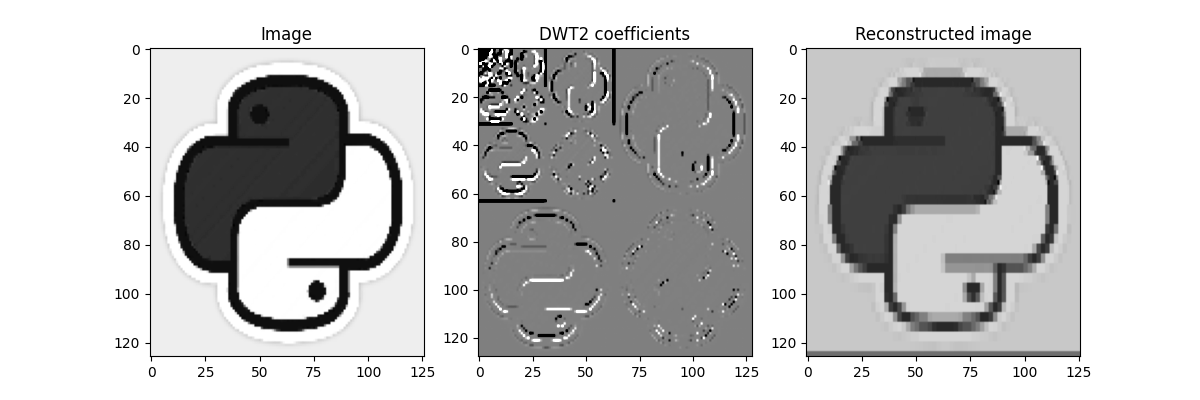# Wavelet transform¶

This example shows how to use the pylops.DWT and pylops.DWT2D operators to perform 1- and 2-dimensional DWT.

import matplotlib.pyplot as plt
import numpy as np

import pylops

plt.close("all")


Let’s start with a 1-dimensional signal. We apply the 1-dimensional wavelet transform, keep only the first 30 coefficients and perform the inverse transform.

nt = 200
dt = 0.004
t = np.arange(nt) * dt
freqs = [10, 7, 9]
amps = [1, -2, 0.5]
x = np.sum([amp * np.sin(2 * np.pi * f * t) for (f, amp) in zip(freqs, amps)], axis=0)

Wop = pylops.signalprocessing.DWT(nt, wavelet="dmey", level=5)
y = Wop * x
yf = y.copy()
yf[25:] = 0
xinv = Wop.H * yf

plt.figure(figsize=(8, 2))
plt.plot(y, "k", label="Full")
plt.plot(yf, "r", label="Extracted")
plt.title("Discrete Wavelet Transform")

plt.figure(figsize=(8, 2))
plt.plot(x, "k", label="Original")
plt.plot(xinv, "r", label="Reconstructed")
plt.title("Reconstructed signal")

••Out:

Text(0.5, 1.0, 'Reconstructed signal')


We repeat the same procedure with an image. In this case the 2-dimensional DWT will be applied instead. Only a quarter of the coefficients of the DWT will be retained in this case.

im = np.load("../testdata/python.npy")[::5, ::5, 0]

Nz, Nx = im.shape
Wop = pylops.signalprocessing.DWT2D((Nz, Nx), wavelet="haar", level=5)
y = Wop * im.ravel()
yf = y.copy()
yf[len(y) // 4 :] = 0
iminv = Wop.H * yf
iminv = iminv.reshape(Nz, Nx)

fig, axs = plt.subplots(1, 3, figsize=(12, 4))
axs.imshow(im, cmap="gray")
axs.set_title("Image")
axs.axis("tight")
axs.imshow(y.reshape(Wop.dimsd), cmap="gray_r", vmin=-1e2, vmax=1e2)
axs.set_title("DWT2 coefficients")
axs.axis("tight")
axs.imshow(iminv, cmap="gray")
axs.set_title("Reconstructed image")
axs.axis("tight")Out:

(-0.5, 125.5, 125.5, -0.5)


Total running time of the script: ( 0 minutes 0.448 seconds)

Gallery generated by Sphinx-Gallery